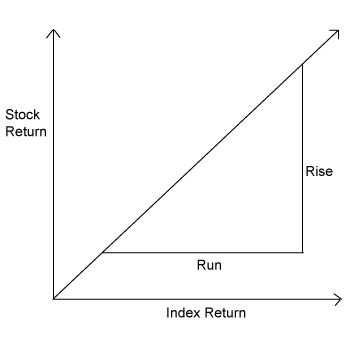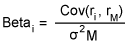## Analysis Intermediate: Evaluating Risk# Beta Compares Investment Risk to the Market

Beta measures the volatility of a security compared to that of the overall market. Beta is the degree to which the returns on an investment will move with the market. It is usually measured against a market index, such as the Standard & Poor’s 500.

### Things To Know

• Beta measures volatility.
• A beta of 1 means that an investment behaves as the market does.

## How it is calculated

Beta is usually calculated for a three-year period. To calculate beta, monthly returns for the security and the benchmark index are plotted on a graph. A line that comes closest to the plotted points is then drawn. The slope (rise/run) of the line gives you your beta.Beta can also be calculated by using the following formula:## What beta numbers mean

A beta of 1 means that the investment behaves as the market does. A beta of more than 1 means the investment is more volatile than the market. A beta of less than 1 means the investment is less volatile than the market.

When the market changes, a security is likely to move at the rate of its beta multiplied by the percentage of the market move. For example, if a stock’s beta is 2 and the market rises by 10 percent, the stock’s returns should rise by 20 percent. If the market falls by 10 percent, the stock’s price should fall by 20 percent.